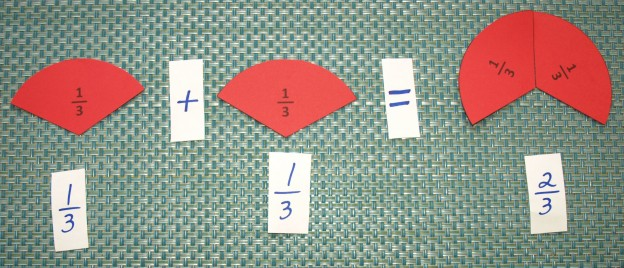# Fraction Circles – Manipulatives for Fractions up to Tenths

The fraction circles are intended to be used as manipulatives to introduce fractions up to tenths. Use the fraction circles to teach addition and subtraction using common denominators, equivalent fractions (to tenths), multiplication and division of fractions, and all operations using mixed numbers.

In addition to using these as manipulatives, the fraction circles can be printed for use as references that can be glued into a math notebook.

What’s Included

• Blank fraction circles (4” in diameter, up to tenths)
• Fraction circles, labeled with the numeric value of each fraction piece (4” in diameter, up to tenths)

**Note: These are all provided in black and white. Please print on colored paper for colored pieces.